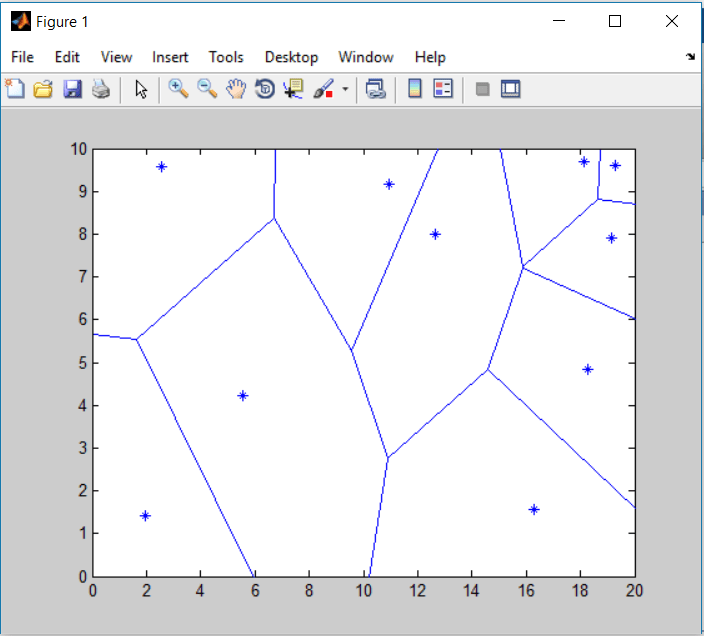In mathematics, a Voronoi Diagram is a partitioning of a plane into regions based on distance to points in a specific subset of the plane.

A Voronoi diagram is defined to be the set of points equidistant from two or more generators (points, segments, polygons, …) under the appropriate metric (usually the Euclidean distance). This construction received considerable attention in the early eighties as a useful tool for motion planning). The main advantage of using the Voronoi diagrams based on the measure of distance is that they have a lower algebraic complexity than those resulting from using the Euclidean distance. These diagrams are piecewise linear for fixed orientations of the moving platform while standard Voronoi diagrams would contain quadratic sheets.

These simplified Voronoi diagrams are sometimes also called straight skeletons.

Despite this important simplification, they still have an important property: any path through free space which starts and ends on the diagram can be continuously deformed so that it lies entirely on the diagram. Thus, they are complete for motion planning, i.e., searching the original space for paths can be reduced to a search on the diagram. Now, to find a path between two points in free space, it suffices to find a path for each point onto the diagram, and to join these points with a path that lies wholly on the diagram.